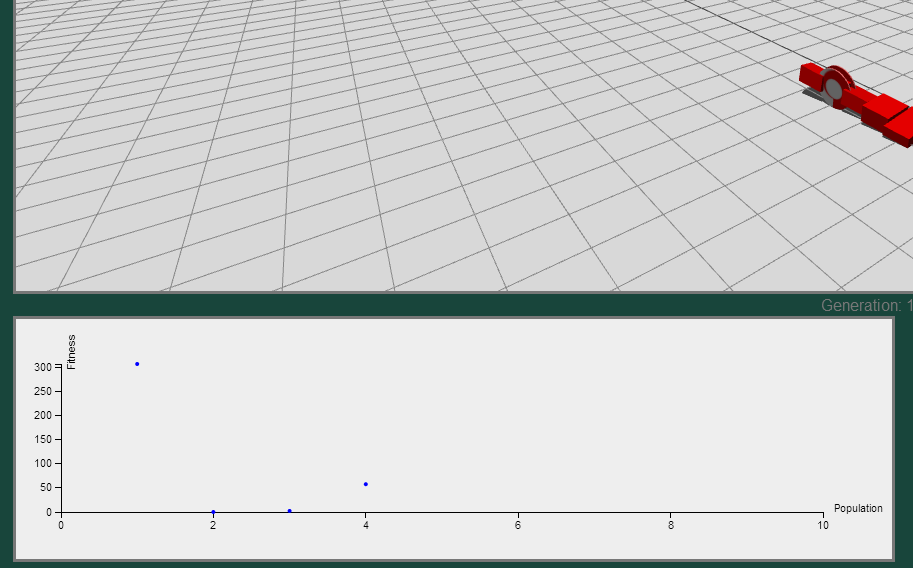# D3.js for Evolutionary Plotting: Animated Scatterplot

D3.js is a Javascript library that allows for data driven documents, according to the website http://d3js.org/.  You can find an astounding number of examples using D3.js covering a wide variety of topics.  However, when building the Evolve-a-Robot website, one of our central goals was to communicate the evolutionary process live.  This meant that rather than generate plots after evolution had completed, as is often the case for offline evolutionary experiments, we wanted instead to connect the live simulation of a robot with its performance in the population.The initial scatterplot conveys the fitness of each individual in a population only after the simulation has concluded.

# Getting iPython Notebook to Run “Correctly” in Mac OS X 10.8

I’m going to keep this post brief so that the steps are clear and concise.  The reason for writing this post is that I wanted to get iPython Notebook, a powerful tool for data analysis, to run with plotting and pandas in Mac OS X 10.8.  When I initially tried to get this running, I would encounter errors where there were conflicts between 32-bit and 64-bit installations of different packages.  After a good deal of trial and error, I found the following steps resulted in a full iPython Notebook environment with Pandas and Matplotlib functioning flawlessly.

# R Quick Tip: Use %in% to filter a data frame.

Working with R, I was looking for functionality to easily subset my data based on a sequence of numbers.  After writing a for loop and using `rbind` to do it initially (terrible to do in R!), I finally found a way to do this efficiently.  Using a command called `%in%`, you can easily apply it as a filter in the `subset` command to get data filtered based on your sequence.  Enjoy!

```# Generate sample data based to test.
sample_data <- data.frame(ID=seq(1,100,1),
Score=sample(0:100,100,rep=TRUE))
summary(sample_data)

# Plot the scores, see that there is a score for each id.
plot(sample_data\$Score~sample_data\$ID)

# Create a filter to apply.
look_at <- seq(1,100,10)

# Filter the sample data by look_at using the %in% command.
subset_data <- subset(sample_data, ID %in% look_at)

# Plot the scores, note the filtered data.
plot(subset_data\$Score~subset_data\$ID)
```

# Starting Quirks with Pandas from an R Junkie

Okay, okay, the title might be a little sensationalised.  I have been using the R statistics package for processing the results of evolutionary runs since beginning my PhD 2 years ago.  In that time, I have become familiar with the basic process to importing data, performing basic population statistics, mean, confidence intervals, etc, and plotting using ggplot.  I’ve always felt that I could streamline the process though as I perform a great deal of preprocessing using Python.  This typically involves combining multiple replicate runs into one data file and possibly even doing some basic statistics using the built-in functionality of Python.